# 更新应用以使用垃圾内容过滤机器学习模型

## 前提条件

• 此 Codelab 面向刚接触机器学习的资深开发者。
• 此 Codelab 是有序衔接课程的一部分。如果您尚未构建基本即时通讯样式应用或构建垃圾评论机器学习模型，请立即停止并执行此操作。

## 您将 [构建或学习]的内容

• 您将学习如何将自定义模型集成到上一步中创建的应用。

## 2. 打开现有 Android 应用

```git clone https://github.com/googlecodelabs/odml-pathways
```

finished 代码也以 `TextClassificationStep2` 的形式提供给您。

## 3.导入模型文件和元数据

1. 使用项目导航器，确保在顶部选择了 Android
2. 右键点击 app 文件夹。依次选择新建 > 目录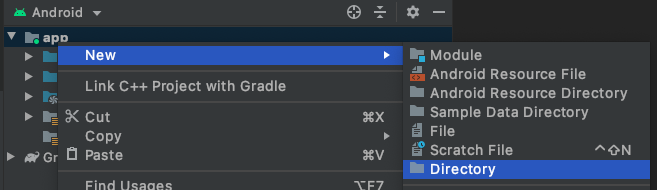1. New Directory 对话框中，选择 src/main/assets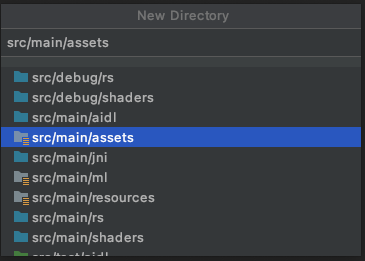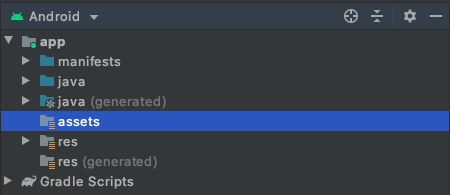1. 右键点击 assets.
2. 在打开的菜单中，您会看到（在 Mac 上）在 Finder 中显示。选择它。（在 Windows 上，系统会显示在资源管理器中显示；而在 Ubuntu 上，则会显示在文件中显示）。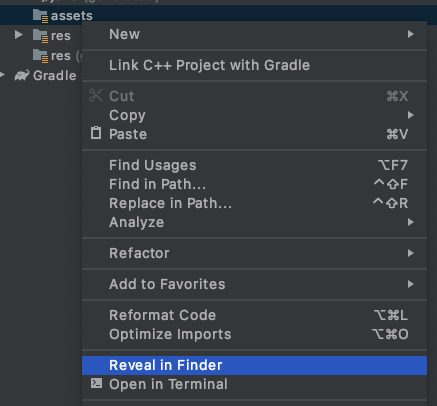1. `labels.txt``model.tflite``vocab` 文件复制到此目录。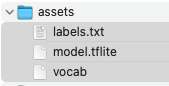1. 返回 Android Studio，您将在 assets 文件夹中看到这些素材资源。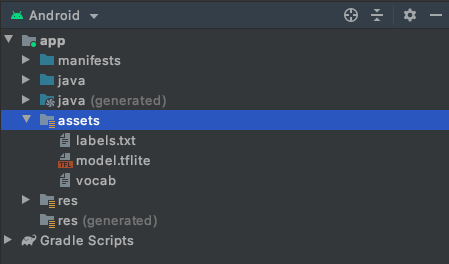## 4.更新您的 build.gradle 以使用 TensorFlow Lite

Android 项目通常有多个级别，因此请务必找到应用级别 1。在 Android 视图中的项目资源管理器中，找到 Gradle Scripts 部分。正确的请求将带有 .app 标签，如下所示：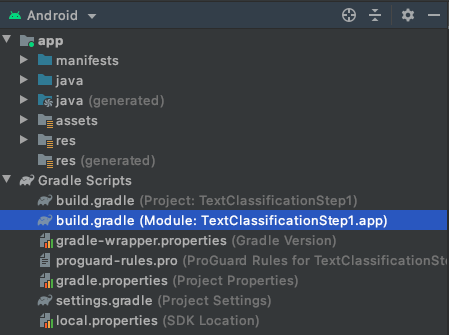``````implementation 'org.tensorflow:tensorflow-lite-task-text:0.1.0'
``````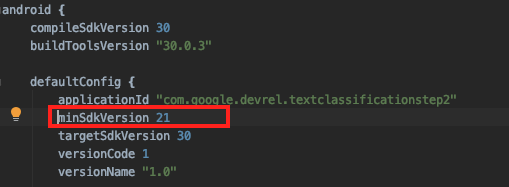## 5. 添加帮助程序类

1. 右键点击 `MainActivity` 代码所在的软件包名称。
2. 依次选择 New > Package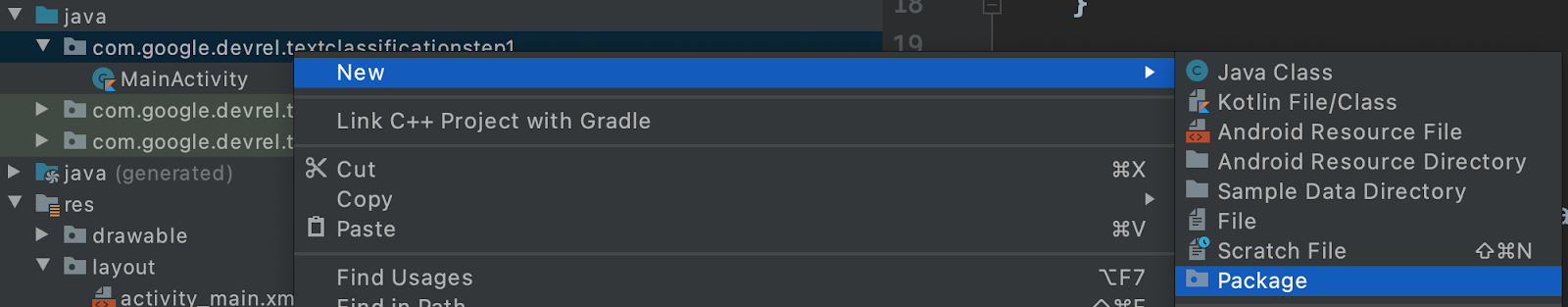1. 屏幕中央会显示一个对话框，要求您输入软件包名称。将它添加到当前软件包名称的末尾。（此处称为帮助程序）。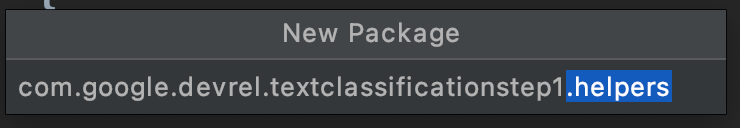1. 完成此操作后，在 Project Explorer 中右键点击 helpers 文件夹。
2. 依次选择 New > Java Class，并将其命名为 `TextClassificationClient`。您将在下一步中编辑该文件。

``````package com.google.devrel.textclassificationstep1.helpers;

public class TextClassificationClient {
}
``````
1. 使用以下代码更新文件：
``````package com.google.devrel.textclassificationstep2.helpers;

import android.content.Context;
import android.util.Log;
import java.io.IOException;
import java.util.List;

import org.tensorflow.lite.support.label.Category;

public class TextClassificationClient {
private static final String MODEL_PATH = "model.tflite";
private static final String TAG = "CommentSpam";
private final Context context;

NLClassifier classifier;

public TextClassificationClient(Context context) {
this.context = context;
}

public void load() {
try {
classifier = NLClassifier.createFromFile(context, MODEL_PATH);
} catch (IOException e) {
Log.e(TAG, e.getMessage());
}
}

public void unload() {
classifier.close();
classifier = null;
}

public List<Category> classify(String text) {
List<Category> apiResults = classifier.classify(text);
return apiResults;
}

}
``````

`load()` 方法中，它会从模型路径实例化一个新的 `NLClassifier` 类型。模型路径只是模型名称 `model.tflite``NLClassifier` 类型是文本任务库的一部分，它可以帮助您将字符串转换为令牌、使用正确的序列长度，将其传递给模型并解析结果。

（如需了解详情，请再次参阅“垃圾评论”机器学习模型。）

## 6.对文本进行分类

`MainActivity` 中，您首先要导入刚刚创建的帮助程序！

1. `MainActivity.kt` 的顶部，与其他导入项一起添加：
``````import com.google.devrel.textclassificationstep2.helpers.TextClassificationClient
import org.tensorflow.lite.support.label.Category
``````
1. 接下来，您需要加载帮助程序。在 `onCreate` 中的 `setContentView` 代码行后面，添加以下几行代码以实例化并加载帮助程序类：
``````val client = TextClassificationClient(applicationContext)
``````

``````btnSendText.setOnClickListener {
var toSend:String = txtInput.text.toString()
txtOutput.text = toSend
}
``````
1. 请按如下所示进行更新：
``````btnSendText.setOnClickListener {
var toSend:String = txtInput.text.toString()
var results:List<Category> = client.classify(toSend)
val score = results.score
if(score>0.8){
txtOutput.text = "Your message was detected as spam with a score of " + score.toString() + " and not sent!"
} else {
txtOutput.text = "Message sent! \nSpam score was:" + score.toString()
}
txtInput.text.clear()
}
``````

1. 以下代码行会将用户输入的字符串传递给模型，从而获得结果：
``````var results:List<Category> = client.classify(toSend)
``````

（TensorFlow 按字母顺序对其进行排序，因此 False 将是项 0，True 将是项 1。）

1. 如需获取值为 `True` 的概率的得分，您可以查看 results.score，如下所示：
``````    val score = results.score
``````
1. 选择了阈值（在本例中为 0.8），您说如果 True 类别的得分高于阈值 (0.8)，则邮件为垃圾邮件。否则，这不是垃圾邮件，且邮件可以安全发送：
``````    if(score>0.8){
txtOutput.text = "Your message was detected as spam with a score of " + score.toString() + " and not sent!"
} else {
txtOutput.text = "Message sent! \nSpam score was:" + score.toString()
}
``````
1. 在此处查看该模型的实际运用。“访问我的博客以买东西！”消息已标记为疑似垃圾内容的可能性：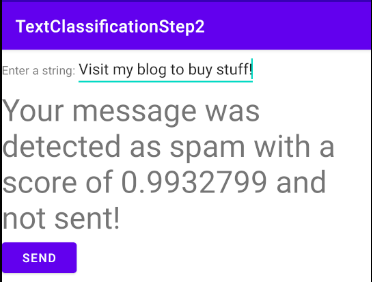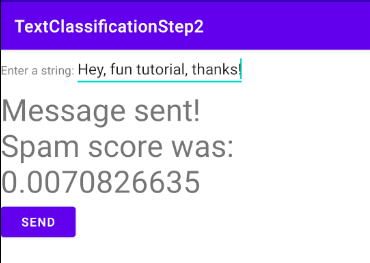## 7. 更新您的 iOS 应用以使用 TensorFlow Lite 模型

finished 代码也以 `TextClassificationStep2` 的形式提供给您。

1. 安装 CocoaPods 后，在 TextClassification 应用的 `.xcproject` 目录下创建一个名为 Podfile 的文件。此文件的内容应如下所示：
``````target 'TextClassificationStep2' do
use_frameworks!

# Pods for NLPClassifier
pod 'TensorFlowLiteSwift'

end
``````

## 8. 添加模型和 Vocab 文件

1. 将它们从 Finder 拖放到您的项目窗口中，即可将它们添加到您的项目中。确保已选中添加到目标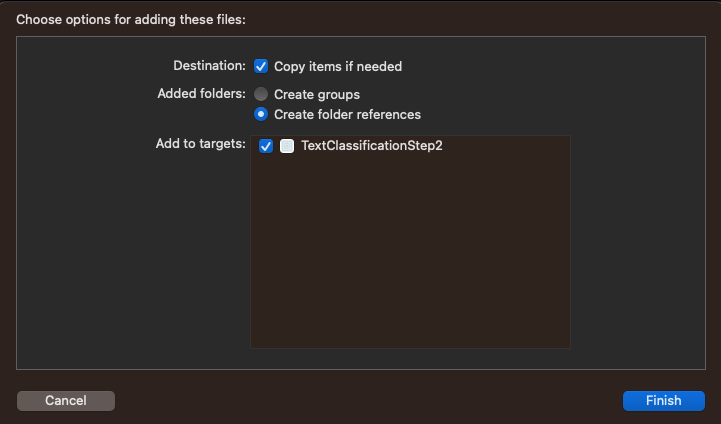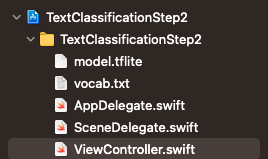1. 仔细检查您的项目是否已添加到 bundle 中（以便将其部署到设备），方法是选择您的项目（在上面的屏幕截图中，它是蓝色图标 TextClassificationStep2），然后查看 Build Phases 标签页：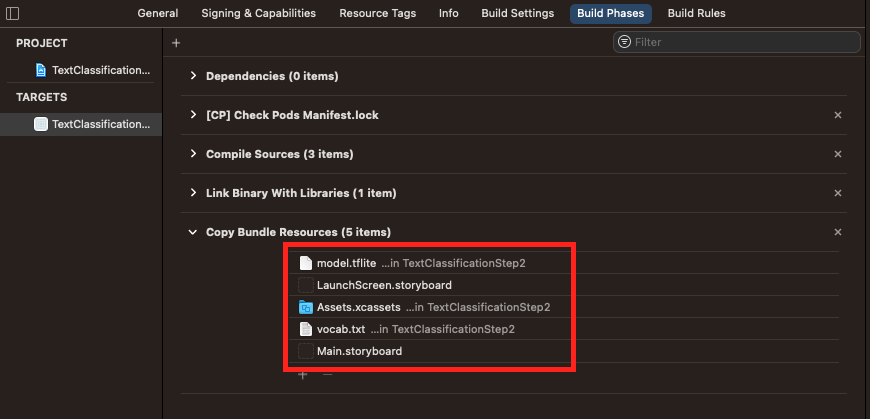## 9. 加载词汇表

1. 定义用于存储字典的类级变量：
``````var words_dictionary = [String : Int]()
``````
1. 然后，在类中创建一个 `func`，以将词汇表加载到此字典中：
``````func loadVocab(){
// This func will take the file at vocab.txt and load it into a has table
// called words_dictionary. This will be used to tokenize the words before passing them
// to the model trained by TensorFlow Lite Model Maker
if let filePath = Bundle.main.path(forResource: "vocab", ofType: "txt") {
do {
let dictionary_contents = try String(contentsOfFile: filePath)
let lines = dictionary_contents.split(whereSeparator: \.isNewline)
for line in lines{
let tokens = line.components(separatedBy: " ")
let key = String(tokens)
let value = Int(tokens)
words_dictionary[key] = value
}
} catch {
print("Error vocab could not be loaded")
}
} else {

}
}
``````
1. 您可以通过在 `viewDidLoad` 内调用它来运行它：
``````override func viewDidLoad() {
txtInput.delegate = self
}
``````

## 10. 将字符串转换为令牌序列

NLP 模型通常接受固定的序列长度。使用 `ragged tensors` 构建的模型也有例外情况，但在大多数情况下，您会发现此问题已得到修复。您在创建模型时指定了该长度。请务必在 iOS 应用中使用相同的长度。

``````let SEQUENCE_LENGTH = 20
``````

``````func convert_sentence(sentence: String) -> [Int32]{
// This func will split a sentence into individual words, while stripping punctuation
// If the word is present in the dictionary it's value from the dictionary will be added to
// the sequence. Otherwise we'll continue

// Initialize the sequence to be all 0s, and the length to be determined
// by the const SEQUENCE_LENGTH. This should be the same length as the
// sequences that the model was trained for
var sequence = [Int32](repeating: 0, count: SEQUENCE_LENGTH)
var words : [String] = []
sentence.enumerateSubstrings(
in: sentence.startIndex..<sentence.endIndex,options: .byWords) {
(substring, _, _, _) -> () in words.append(substring!) }
var thisWord = 0
for word in words{
if (thisWord>=SEQUENCE_LENGTH){
break
}
let seekword = word.lowercased()
if let val = words_dictionary[seekword]{
sequence[thisWord]=Int32(val)
thisWord = thisWord + 1
}
}
return sequence
}
``````

## 11. 进行分类

``````import TensorFlowLite
``````

``````func classify(sequence: [Int32]){
// Model Path is the location of the model in the bundle
let modelPath = Bundle.main.path(forResource: "model", ofType: "tflite")
var interpreter: Interpreter
do{
interpreter = try Interpreter(modelPath: modelPath!)
} catch _{
return
}
``````

``````let tSequence = Array(sequence)
let myData = Data(copyingBufferOf: tSequence.map { Int32(\$0) })
let outputTensor: Tensor
``````

``````do {
// Allocate memory for the model's input `Tensor`s.
try interpreter.allocateTensors()

// Copy the data to the input `Tensor`.
try interpreter.copy(myData, toInputAt: 0)

// Run inference by invoking the `Interpreter`.
try interpreter.invoke()
``````

``````// Get the output `Tensor` to process the inference results.
outputTensor = try interpreter.output(at: 0)
// Turn the output tensor into an array. This will have 2 values
// Value at index 0 is the probability of negative sentiment
// Value at index 1 is the probability of positive sentiment
let resultsArray = outputTensor.data
let results: [Float32] = [Float32](unsafeData: resultsArray) ?? []
``````

``````let positiveSpamValue = results
var outputString = ""
if(positiveSpamValue>0.8){
outputString = "Message not sent. Spam detected with probability: " + String(positiveSpamValue)
} else {
outputString = "Message sent!"
}
txtOutput.text = outputString
``````

``````func classify(sequence: [Int32]){
// Model Path is the location of the model in the bundle
let modelPath = Bundle.main.path(forResource: "model", ofType: "tflite")
var interpreter: Interpreter
do{
interpreter = try Interpreter(modelPath: modelPath!)
} catch _{
Return
}

let tSequence = Array(sequence)
let myData = Data(copyingBufferOf: tSequence.map { Int32(\$0) })
let outputTensor: Tensor
do {
// Allocate memory for the model's input `Tensor`s.
try interpreter.allocateTensors()

// Copy the data to the input `Tensor`.
try interpreter.copy(myData, toInputAt: 0)

// Run inference by invoking the `Interpreter`.
try interpreter.invoke()

// Get the output `Tensor` to process the inference results.
outputTensor = try interpreter.output(at: 0)
// Turn the output tensor into an array. This will have 2 values
// Value at index 0 is the probability of negative sentiment
// Value at index 1 is the probability of positive sentiment
let resultsArray = outputTensor.data
let results: [Float32] = [Float32](unsafeData: resultsArray) ?? []

let positiveSpamValue = results
var outputString = ""
if(positiveSpamValue>0.8){
outputString = "Message not sent. Spam detected with probability: " +
String(positiveSpamValue)
} else {
outputString = "Message sent!"
}
txtOutput.text = outputString

} catch let error {
print("Failed to invoke the interpreter with error: \(error.localizedDescription)")
}
}
``````

## 12. 添加 Swift 扩展程序

``````extension Data {
/// Creates a new buffer by copying the buffer pointer of the given array.
///
/// - Warning: The given array's element type `T` must be trivial in that it can be copied bit
///     for bit with no indirection or reference-counting operations; otherwise, reinterpreting
///     data from the resulting buffer has undefined behavior.
/// - Parameter array: An array with elements of type `T`.
init<T>(copyingBufferOf array: [T]) {
self = array.withUnsafeBufferPointer(Data.init)
}
}
``````

``````extension Array {
/// Creates a new array from the bytes of the given unsafe data.
///
/// - Warning: The array's `Element` type must be trivial in that it can be copied bit for bit
///     with no indirection or reference-counting operations; otherwise, copying the raw bytes in
///     the `unsafeData`'s buffer to a new array returns an unsafe copy.
/// - Note: Returns `nil` if `unsafeData.count` is not a multiple of
///     `MemoryLayout<Element>.stride`.
/// - Parameter unsafeData: The data containing the bytes to turn into an array.
init?(unsafeData: Data) {
guard unsafeData.count % MemoryLayout<Element>.stride == 0 else { return nil }
#if swift(>=5.0)
self = unsafeData.withUnsafeBytes { .init(\$0.bindMemory(to: Element.self)) }
#else
self = unsafeData.withUnsafeBytes {
.init(UnsafeBufferPointer<Element>(
start: \$0,
count: unsafeData.count / MemoryLayout<Element>.stride
))
}
#endif  // swift(>=5.0)
}
}
``````

## 13. 运行 iOS 应用## 14. 恭喜！

[{ "type": "thumb-down", "id": "missingTheInformationINeed", "label":"没有我需要的信息" },{ "type": "thumb-down", "id": "tooComplicatedTooManySteps", "label":"太复杂/步骤太多" },{ "type": "thumb-down", "id": "outOfDate", "label":"内容需要更新" },{ "type": "thumb-down", "id": "translationIssue", "label":"翻译问题" },{ "type": "thumb-down", "id": "samplesCodeIssue", "label":"示例/代码问题" },{ "type": "thumb-down", "id": "otherDown", "label":"其他" }]
[{ "type": "thumb-up", "id": "easyToUnderstand", "label":"易于理解" },{ "type": "thumb-up", "id": "solvedMyProblem", "label":"解决了我的问题" },{ "type": "thumb-up", "id": "otherUp", "label":"其他" }]